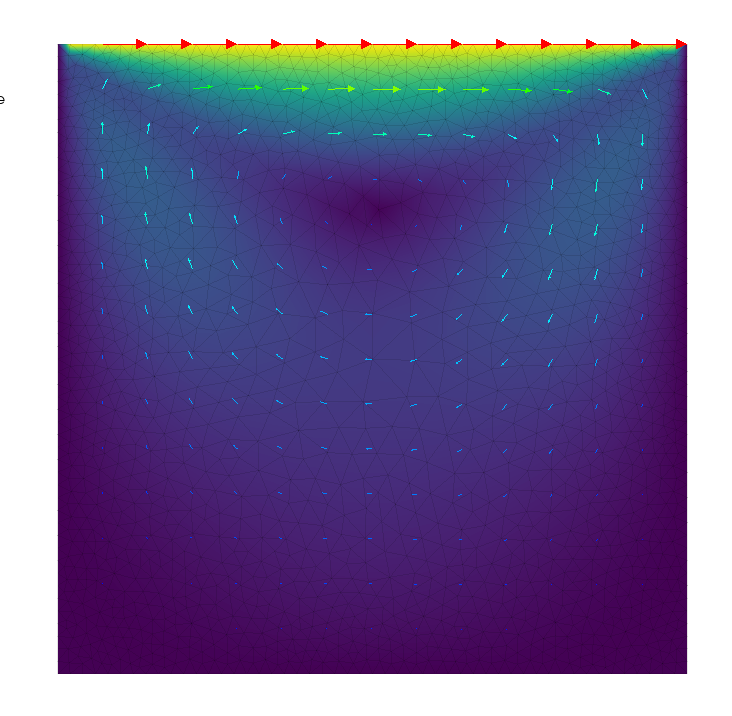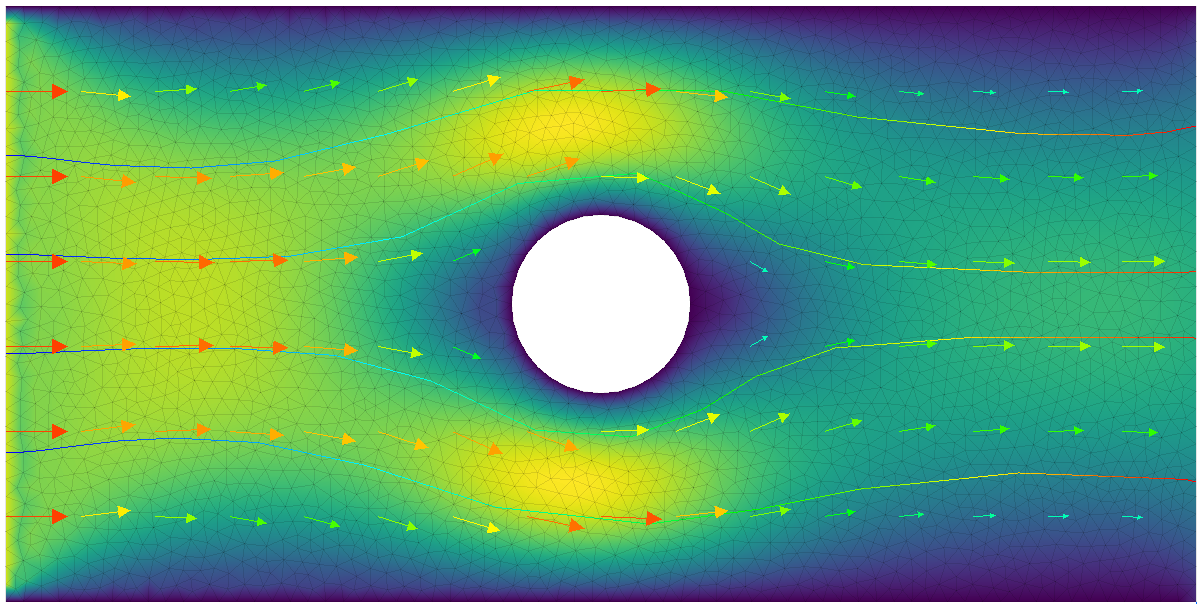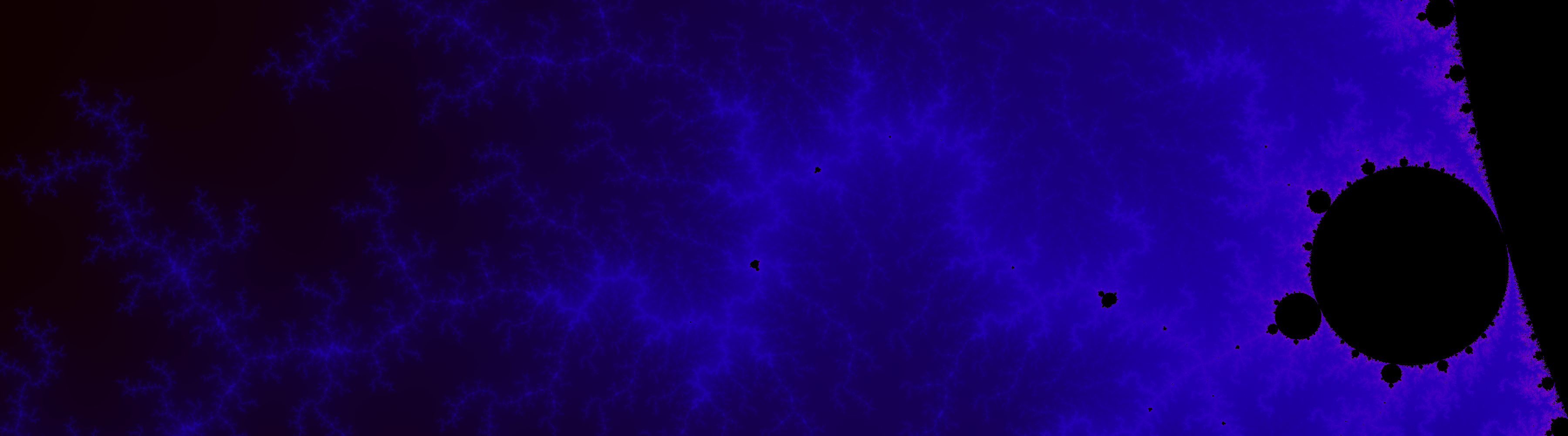### Nonlinear Elasticity

Finite element simulation of a hyperelastic material with nonlinear Kirchhoff-St. Venant constitutive law. The material is stretched upwards for one second and then released inducing the free vibration shown. Roundoff errors eventually build enough to perturb the material laterally.

### Stokes Flow

P2P1 triangles were used to discretize the Stokes Equations.

### Lid Driven CavityThe velocity magnitude and vectors for the Lid Driven Cavity problem. The top wall has a fixed velocity of 1 and the sides and bottom have no slip boundary conditions.

### Flow Over a CylinderThe velocity magnitude, vectors, and streamlines for Stokes Flow across a cylinder.

The code can be found here. Building the stokes.cpp executable and running:
 ./stokes.exe cavity
and
 ./stokes.exe flow
will generate the data for the plots shown above.

### Wave Equation

My arbitrary order FEM code (found here) was used to solve the Wave Equation with dissipation:
$$\frac{\partial^2 u}{\partial t^2} + a \frac{\partial u}{\partial t} = c \nabla^2 u \,,$$
where $$u$$ is the $$z$$ displacement of the wave. $$a$$ controls the dissipation of the wave over time and $$c$$ controls the wave propogation speed. The second derivative in time was handled with a finite difference scheme:
$$\frac{\partial^2 u}{\partial t^2} \approx \frac{u_{i+1} – 2u_i + u_{i-1}}{\Delta t^2} \,.$$
Standard time integration procedures can now be applied.

In the video below, the second order Crank-Nicholson method and a fourth order FEM discretizations were used for space and time, respectively. The video shows the relaxation of the initial sinusoidal perturbation over time in an L shaped domain.

### Mandelbrot

The Mandelbrot set is the set of complex numbers, $$c$$, such that the series
$$z_{n+1} = z_n^2 + c$$
does not diverge as $$n\rightarrow \infty$$. These values of $$c$$ can then be plotted in the complex plane to produce some interesting images. As each pixel in the image is an independent calculation this is a good problem for getting familiar with parallel computing. The following images were generated with the code at https://github.com/smsolivier/Mandelbrot.